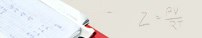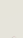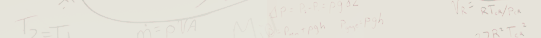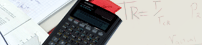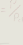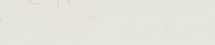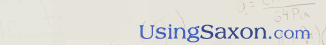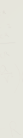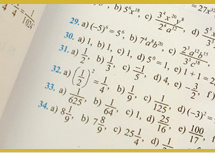Contents of the Algebra 1, 3rd Edition DVD Series (120 Lessons)Introduction: Prerequisites for the Course - How To Use The Math Book - Expectations of Students. Lesson 1: Review of Adding & Subtracting with Fractions & Whole Numbers - Using Line Segments as Fractional Parts of a Line. Lesson 2: The Language of Geometry - Angles, Triangles, Polygons, and Quadrilaterals. Lesson 3: Definition and Application of Perimeter and Circumference. Lesson 4: Review of Basic Arithmetic and the Symbols and Algorithms Used in the Computations. Lesson 5: Composition of the Sets of Numbers - Defining the Absolute Value of Numbers. Lesson 6: Rules for Addition and Subtraction - Using the Absolute Value in Addition & Subtraction. Lesson 7: The "Double Negative" - Using Symbols of Inclusion. Lesson 8: Calculating Areas of Rectangles, Trapezoids, Triangles, Circles & Semi-Circles. Lesson 9: Rules for Multiplications & Division - Working with Inverse Operations. Lesson 10: Multiplying and Dividing by Zero - Properties of Multiple Unit Conversions. Lesson 11: Using Reciprocals - Basic Rules for Order of Operations - Recognizing Addition Patterns from Multiplication Patterns. Lesson 12: Using Symbols of Inclusion in Order of Operations. Lesson 13: Working with Multiple "Symbols of Inclusion" in "Order of Operations" - More on the Double Negative! Lesson 14: Evaluating Algebraic Expressions using Variables and Substitution. Lesson 15: Computing Surface Areas of Right Geometric Solids. Lesson 16: Working with More Advanced Evaluations Involving Substitutions. Lesson 17: Defining Factors, Coefficients, and Terms - Review of the Distributive Property in Multiplication. Lesson 18: Reviewing the Definition of "Like Terms" and then applying that definition to adding "Like Terms." Lesson 19: Evaluating Terms Containing Exponents, Powers, and Roots. Lesson 20: Finding the Volume of Right Geometric Solids. Lesson 21: Defining the "Product Rule" for Exponents - Adding Like Terms that have Exponents. Lesson 22: Review of Definitions for Algebraic Symbols, Words, Statements and Sentences - Defining "Equations." Lesson 23: Defining "Equivalent Equation" - Applying the "Additive Property of Equality" to Equations. Lesson 24: Reviewing the Multiplicative Property of Equality. Lesson 25: Solving an Equation for its unknown Variable. Lesson 26: Working with More Challenging Equations. Lesson 27: Simplifying Equations Containing Decimals - Advanced work with the "Distributive Property." Lesson 28: Word Problems Involving Fractions - Applying Functional Notation. Lesson 29: Rules for Working with Terms Containing Negative and Zero Exponents. Lesson 30: Defining and Using Algebraic Phrases - Word Problems Involving Decimal Parts of Numbers. Lesson 31: Working with Equations Containing Symbols of Inclusion (Parenthesis). Lesson 32: Word Problems Using Algebraic Phrases. Lesson 33: Working with Products of Prime Factors - Word Problems containing Statements of Unequal Quantities. Lesson 34: Finding the Greatest Common Factor (GCF). Lesson 35: Factoring using the Greatest Common Factor (GCF) - Proper use of Factoring in Algebraic Terms Lesson 36: Using the Distributive Property with Positive Exponents - Recognizing a Negative Exponent from a Negative Number. Lesson 37: Defining and Graphing Symbols of Inequality. Lesson 38: Defining and Working with Ratios and Proportions Lesson 39: More on Symbols of Inequality - Defining & Applying Trichotomy - Working with Word Problems Involving Ratios. Lesson 40: Working with Negative Exponents - Applying the Distributive Property of Equality to Negative Exponents. Lesson 41: Advanced Application of Adding Like Terms - Using Substitution in Evaluating Equations. Lesson 42: Working with Equations Containing More than one Variable. Lesson 43: Defining and Working with Least Common Multiples in Algebraic Expressions. Lesson 44: Addition of Rational Expressions with identical and with different Denominators. Lesson 45: Definition and Application of the Mean, Median and Mode Lesson 46: Definition and Application of a mathematical Conjunction Lesson 47: Evaluating Percents - Less Than - And Greater Than - 100% Lesson 48: Defining and Working with Polynomials of Varying Degrees. Lesson 49: Applying the Distributive Property to the Multiplication of Polynomials. Lesson 50: More Work with Polynomials - Defining an "Ordered Pair" on the Cartesian Coordinate System. Lesson 51: Graphing Linear Equations using the Cartesian Coordinate System. Lesson 52: Advanced Rational Expressions using Unequal Denominators - Determining "Overall" Average. Lesson 53: Applying the "Power Rule" to Exponents - Using Unit Multipliers for converting Volume. Lesson 54: Using Substitution to solve for Unknown Variables in Equations with more than One Variable. Lesson 55: Establishing Rules for Working with Abstract, Complex Fractions. Lesson 56: Defining finite and infinite sets of numbers - Advanced Linear Graphing Techniques. Lesson 57: Adding Algebraic Terms Containing Negative Exponents. Lesson 58: Advanced Word Problems dealing with Percents. Lesson 59: Advanced Techniques for solving Two Unknown with Two Equations. Lesson 60: Finding Volume and Surface Areas of Geometric Solids (Prisms & Cylinders). Lesson 61: Review of the Subsets of Real Numbers - Introduction to The Calculator (Casio fx260 solar - \$9.99) Lesson 62: Determining Higher Order Roots - Evaluating the notation "+/-." Lesson 63: Multiplying radicals - Recognizing Repeating Decimals. Lesson 64: Defining "Domain" - Review of the Additive Property of Equality and its application to the Property of Inequality. Lesson 65: Adding Radical Expressions - Defining "Weighted Averages." Lesson 66: Simplifying Radical Terms or Expressions - Simplifying the Square Roots of Large Numbers Lesson 67: Review of the Definition of an Equation - Solving Multiple Equations using Elimination rather than Substitution. Lesson 68: Advanced Abstract, Complex Fractions. Lesson 69: Introduction to Factoring Trinomials. Lesson 70: Introduction to Probability - Dependency. Lesson 71: Factoring Trinomials with Common Factors - Should We Use Subscripted Variables? Lesson 72: Advanced Factoring in Trinomials - Volume and Surface Area of Pyramids and Cones. Lesson 73: Defining the Difference of Two Squares - More on Probability. Lesson 74: Review and Use of Scientific Notation Lesson 75: Linear Equations - Graphing a Line Using the "Slope-Intercept" method. Lesson 76: Defining "Consecutive Integers." Lesson 77: Defining "Consecutive 'Odd' or 'Even' Integers" - Word Problems using Fractions and Decimals Lesson 78: Solving Equations using "Common Denominators." Lesson 79: Solving Equations containing Multiple Subscripted Variables. Lesson 80: Working with Scientific Notation involving very large or very small Numbers. Lesson 81: The Three Categories of Linear Equations (Consistent, Inconsistent, or Dependent) Lesson 82: Defining and Evaluating Functions - Determining the Domain and Range of a Function. Lesson 83: Word Problems Using Subscripted Variables. Lesson 84: Multiplying Radicals - Mapping and Defining Functions and Their Relations. Lesson 85: Review of "Stem and Leaf" Plots - Using Them to Create Graphs. Lesson 86: Dividing Polynomials. Lesson 87: Solving Systems of Equations using an Algorithm - Using the Vertical Line Test to Determine Functions. Lesson 88: Factoring Quadratic Equations. Lesson 89: Word Problems Dealing with Money or other Values. Lesson 90: Word Problems Utilizing Ratios, and the Multiplication, Division, Addition and Subtraction of Numbers. Lesson 91: Using the Multiplicative Property of Inequality - Volume and Surface Area of Spheres. Lesson 92: Word Problems involving Equal Distances and Uniform Rates. Lesson 93: Factoring with Products of Polynomials - Simplifying Abstract, Complex Fractions. Lesson 94: More Distance Word Problems involving The Sum of Two Different Distances. Lesson 95: Recognizing and Graphing Unusual Non-Linear Functions. Lesson 96: Introduction of the "Difference of 'Two Squares' Theorem." Lesson 97: Re-Visiting the "Pythagorean Theorem" - What is a Pythagorean Triplet. Lesson 98: Using the "Slope Formula" and the "Pythagorean Theorem" to find the Distance between Two Points. Lesson 99: More Distance Word Problems involving Uniform Motion, but comparing Two Unequal Distances Lesson 100: Our "Base Ten" Place Value System - Rules for Rounding Numbers. Lesson 101: Factoring Trinomials in the Denominator & Using these Factors to find a "Common Denominator." Lesson 102: Use of the "Absolute Value" in Statements of "Inequality." Lesson 103: Solving Equations using "Common Denominators" containing "Symbols of Inclusion." Lesson 104: More Abstract, Rational Equations. Lesson 105: Using a Technique called "Grouping" to factor Polynomials. Lesson 106: The "Slope-Intercept Form of Linear Equations - Finding the Equation of a Line Passing Through Two Specific Points. Lesson 107: Finding the Equation of a Line "Passing through a Specific Point", but also "Parallel" to another given line. Lesson 108: Solving Radical Equations, using "Symbols of Inclusion." Lesson 109: Factoring Trinomials using a Different Method ("Factoring over the Integers"). Lesson 110: Shifts and Reflections in the Location of the Vertex of a Non-linear Function Lesson 111: A New Form of Conjunctions - Introduction to Disjunctions. Lesson 112: Advanced Multiplication of Radical Expressions using Double "Symbols of Inclusion." Lesson 113: Introduction to Direct and Inverse Variation. Lesson 114: Exponential Growth. Lesson 115: Graphing Linear "Inequalities." Lesson 116: Rationalizing The Denominator using "The Quotient Rule." Lesson 117: More Word Problems involving Direct and Inverse Variation using "Squared" terms. Lesson 118: Factoring a Trinomial Using a technique called "Completing the Square." Lesson 119: Introduction to the Quadratic Formula. Lesson 120: Re-visiting "Box and Whisker Plots." Home | Review the book | Purchase book | Testimonials | Newsletter | Contact Us © 2006 - 2021 AJ Publishers, LLC